### Polynomials Class 10th Mathematics CBSE Solution

##### Question 1.Verify that the numbers given alongside of the cubic polynomials below are their zeroes. Also verify the relationship between the zeroes and the coefficients in each case:(i)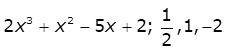(ii)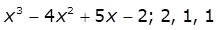Answer:(i) P(x) =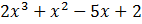Now for zeroes, putting the given values in x.P(1/2) = 2(1/2)3 + (1/2)2 - 5(1/2) + 2= (1/4) + (1/4) - (5/2) + 2= (1 + 1 - 10 + 8)/2= 0/2 = 0P(1) =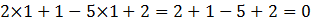P(-2) =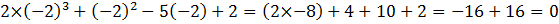Thus, 1/2, 1 and -2 are zeroes of given polynomial.Comparing given polynomial with ax3 + bx2 + cx + d and Taking zeroes as α, β, and γ, we have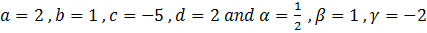Now, We know the relation between zeroes and the coefficient of a standard cubic polynomial as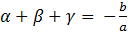Substituting value, we have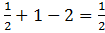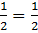Since, LHS = RHS (Relation Verified)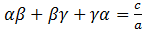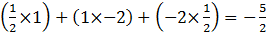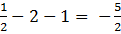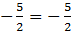Since LHS = RHS, Relation verified.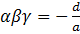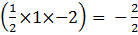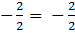Since LHS = RHS, Relation verified.Thus, all three relationships between zeroes and the coefficient is verified.(ii) p(x) = x3 – 4x2 + 5x – 2Now for zeroes , put the given value in x.P(2) =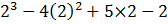=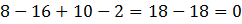P(1) =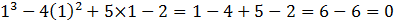P(1) =Thus, 2, 1 , 1 are the zeroes of the given polynomial.Now,Comparing the given polynomial with ax3 + bx2 + cx + d, we get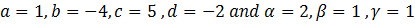Now,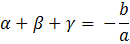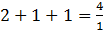4 = 4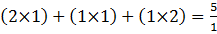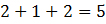5 = 5αβγ =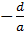2 × 1 × 1 = 22 = 2Thus, all three relationships between zeroes and the coefficient is verified.Question 2.Find a cubic polynomial with the sum, sum of the product of its zeroes taken two at a time, and the product of its zeroes as 2, -7, -14 respectively.Answer:For a cubic polynomial equation, ax3 + bx2 + cx + d, and zeroes α, β and γwe know thatLet the polynomial be ax3 + bx2 + cx + d, and zeroes α, β and γ.A cubic polynomial with respect to its zeroes is given by,x3 - (sum of zeroes) x2 + (Sum of the product of roots taken two at a time) x - Product of Roots = 0x3 - (2) x2 + (- 7) x - (- 14) = 0x3 - (2) x2 + (- 7) x + 14 = 0Hence, the polynomial is x3 - 2x2 - 7x + 14.Question 3.If the zeroes of the polynomial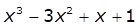are (a - b), a and (a + b). Find a and b.Answer:Given,p(x) =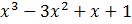zeroes are = a – b , a + b , aC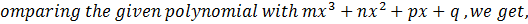=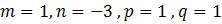Sum of zeroes =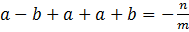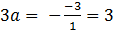a =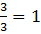The zeroes are = (1 - b), 1 and (1 + b)Product of zeroes = (1 - b)(1 + b)(1 - b)(1 + b) = -q/m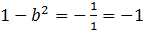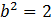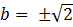So,We get,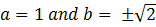Question 4.If two zeroes of the polynomial x4 - 6x3 - 26x2 + 138x - 35 are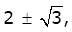find other zeroes.Answer:Given:2+√3 and 2-√3 are zeroes of given equation, Therefore, (x - 2 + √3)(x - 2 - √3) should be a factor of given equation.Also, (x - 2 + √3)(x - 2 - √3) = x2 - 2x - √3x -2x + 4 + 2√3 + √3x - 2√3 - 3= x2 - 4x + 1To find other zeroes, we divide given equation by x2 - 4x + 1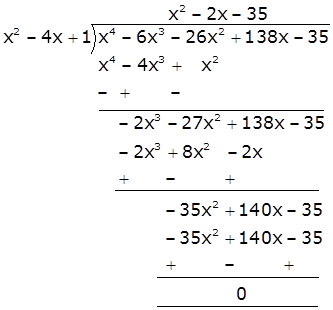We get ,x4 - 6x3 - 26x2 + 138x - 35 = (x2 - 4x + 1)(x2 - 2x - 35)Now factorizing x2 - 2 x - 35 we get,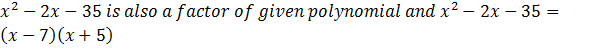The value of polynomial is also zero when ,x - 7 = 0orx = 7And, x + 5 = 0orx = -5Hence, 7 and -5 are also zeroes of this polynomial.Question 5.If the polynomial x4 - 6x3 + 16x2 - 25x + 10 is divided by another polynomial x2 - 2k + k the remainder comes out to be x + a, find k and a. Answer:To solve this question divide x4 - 6 x3 + 16 x2 - 25 x + 10 by x2 - 2 x + k by long division methodLet us divide,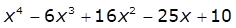by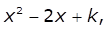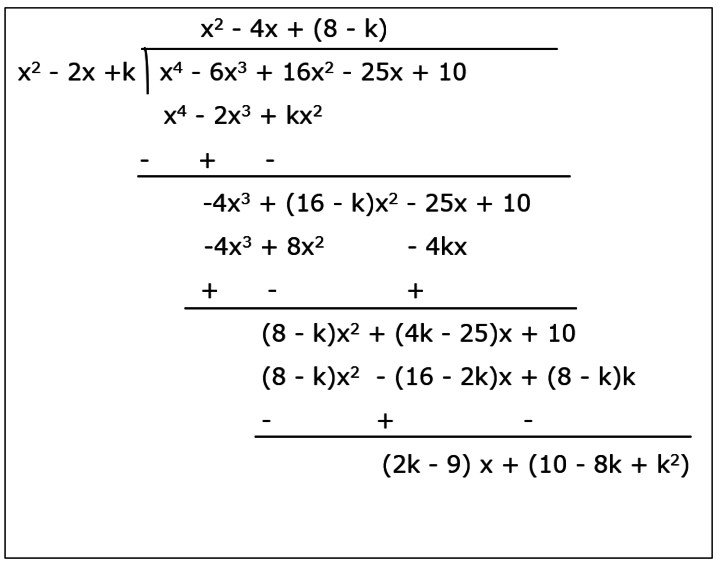So, remainder = (2k - 9)x + (10 - 8k + k2)But given remainder = x + a⇒ (2k - 9)x + (10 - 8k + k2) = x + aComparing coefficient of x, we have2k - 9 = 1⇒ 2k = 10⇒ k = 5andComparing constant term,10 - 8k + k2 = a⇒ a = 10 - 8(5) + 52⇒ a = 10 - 40 + 25⇒ a = -5So, the value of k is 5 and a is -5.

PDF FILE TO YOUR EMAIL IMMEDIATELY PURCHASE NOTES & PAPER SOLUTION. @ Rs. 50/- each (GST extra)

HINDI ENTIRE PAPER SOLUTION

MARATHI PAPER SOLUTION

SSC MATHS I PAPER SOLUTION

SSC MATHS II PAPER SOLUTION

SSC SCIENCE I PAPER SOLUTION

SSC SCIENCE II PAPER SOLUTION

SSC ENGLISH PAPER SOLUTION

SSC & HSC ENGLISH WRITING SKILL

HSC ACCOUNTS NOTES

HSC OCM NOTES

HSC ECONOMICS NOTES

HSC SECRETARIAL PRACTICE NOTES

# 2019 Board Paper Solution

HSC ENGLISH SET A 2019 21st February, 2019

HSC ENGLISH SET B 2019 21st February, 2019

HSC ENGLISH SET C 2019 21st February, 2019

HSC ENGLISH SET D 2019 21st February, 2019

SECRETARIAL PRACTICE (S.P) 2019 25th February, 2019

HSC XII PHYSICS 2019 25th February, 2019

CHEMISTRY XII HSC SOLUTION 27th, February, 2019

OCM PAPER SOLUTION 2019 27th, February, 2019

HSC MATHS PAPER SOLUTION COMMERCE, 2nd March, 2019

HSC MATHS PAPER SOLUTION SCIENCE 2nd, March, 2019

SSC ENGLISH STD 10 5TH MARCH, 2019.

HSC XII ACCOUNTS 2019 6th March, 2019

HSC XII BIOLOGY 2019 6TH March, 2019

HSC XII ECONOMICS 9Th March 2019

SSC Maths I March 2019 Solution 10th Standard11th, March, 2019

SSC MATHS II MARCH 2019 SOLUTION 10TH STD.13th March, 2019

SSC SCIENCE I MARCH 2019 SOLUTION 10TH STD. 15th March, 2019.

SSC SCIENCE II MARCH 2019 SOLUTION 10TH STD. 18th March, 2019.

SSC SOCIAL SCIENCE I MARCH 2019 SOLUTION20th March, 2019

SSC SOCIAL SCIENCE II MARCH 2019 SOLUTION, 22nd March, 2019

XII CBSE - BOARD - MARCH - 2019 ENGLISH - QP + SOLUTIONS, 2nd March, 2019

# HSCMaharashtraBoardPapers2020

(Std 12th English Medium)

HSC ECONOMICS MARCH 2020

HSC OCM MARCH 2020

HSC ACCOUNTS MARCH 2020

HSC S.P. MARCH 2020

HSC ENGLISH MARCH 2020

HSC HINDI MARCH 2020

HSC MARATHI MARCH 2020

HSC MATHS MARCH 2020

# SSCMaharashtraBoardPapers2020

(Std 10th English Medium)

English MARCH 2020

HindI MARCH 2020

Hindi (Composite) MARCH 2020

Marathi MARCH 2020

Mathematics (Paper 1) MARCH 2020

Mathematics (Paper 2) MARCH 2020

Sanskrit MARCH 2020

Sanskrit (Composite) MARCH 2020

Science (Paper 1) MARCH 2020

Science (Paper 2)

Geography Model Set 1 2020-2021

MUST REMEMBER THINGS on the day of Exam

Are you prepared? for English Grammar in Board Exam.

Paper Presentation In Board Exam

How to Score Good Marks in SSC Board Exams

Tips To Score More Than 90% Marks In 12th Board Exam

How to write English exams?

How to prepare for board exam when less time is left

How to memorise what you learn for board exam

No. 1 Simple Hack, you can try out, in preparing for Board Exam

How to Study for CBSE Class 10 Board Exams Subject Wise Tips?

JEE Main 2020 Registration Process – Exam Pattern & Important Dates

NEET UG 2020 Registration Process Exam Pattern & Important Dates

How can One Prepare for two Competitive Exams at the same time?

8 Proven Tips to Handle Anxiety before Exams!